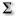# Statistics

This category contains 18 nodes.

##### Bland-Altman Plot

Creates a Bland-Altman plot (also known as Tukey mean-difference plot) used to analyze the agreement of two different measurement methods.

##### Friedman Test

States whether three or more samples show significant statistical difference in their location parameters

##### Kaplan-Meier Estimator

Calculates the Kaplan-Meier estimator for a given data set of study subjects and events at certain time stamps.

##### Kaplan-Meier EstimatorDeprecated

Calculates the Kaplan-Meier estimator for a given data set of study subjects and events at certain time stamps.

##### Kolmogorov-Smirnov Test

States whether two samples come from the same distribution

##### Kruskal-Wallis Test

Performs the Kruskal-Wallis independent group test on multiple groups.

##### Linear Discriminant AnalysisDeprecated

This node performs a linear discriminant analysis.

##### Numeric Median Test

Compares the distributions of two columns to the median of the two columns together, to determine if their distributions are different

##### Odds and Risk Ratios

Calculates the Odds Ratio and Risk Ratio from a 2x2 contingency table.

##### Odds and Risk RatiosDeprecated

Calculates the Odds Ratio and Risk Ratio from a 2x2 contingency table.